# IBDP Past Year Exam Questions – Transformation

Q1.   [N10.P2]

The diagram shows the graph of a linear function  and a quadratic function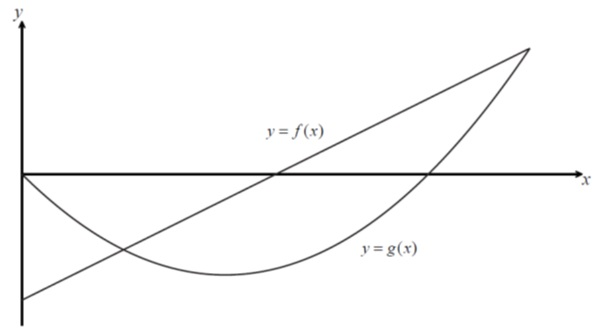On the same axes sketch the graph of $\frac{f}{g}$ . Indicate clearly where the x-intercept and the asymptotes occur.   [5 marks]

Q2.   [M08.P2.TZ2]

The graph of  $y=f\left(x\right)$ for $–2\le x\le 8$ is shown.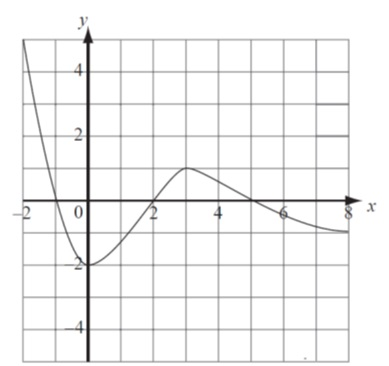On the set of axes provided, sketch the graph of  $y=\frac{1}{f\left(x\right)}$ , clearly showing any asymptotes and indicating the coordinates of any local maxima or minima.     [5marks]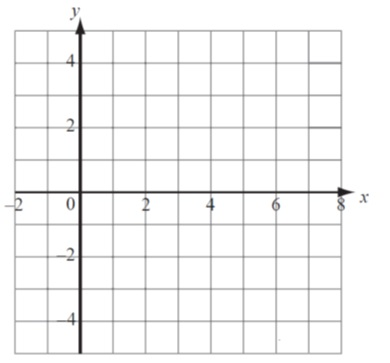Q3.   [M09.P2]

(a) The graph of $y=\mathrm{ln}\left(x\right)$ is transformed into the graph of $y=\mathrm{ln}\left(2x+1\right)$ . Describe two transformations that are required to do this.    [2marks]

(b) Solve .       [4 marks]

Q4.   [M15.P2]

The graph of $y=\mathrm{ln}\left(5x+10\right)$ is obtained from the graph of $y=\mathrm{ln}x$ by a translation of $a$ units in the direction of the xaxis followed by a translation of $b$  units in the direction of the yaxis.

(a) Find the value of $a$  and the value of $b$ .        [4 marks]

Q5.   [M12.P2]

Let $f\left(x\right)=\mathrm{ln}x$ . The graph of $f$  is transformed into the graph of the function $g$  by a translation of  $\left(\begin{array}{c}3\\ –2\end{array}\right)$ , followed by a reflection in the x-axis. Find an expression for $g\left(x\right)$ , giving your answer as a single logarithm.        [5 marks]

Q6.   [N14.P1]

The function f is defined by  . The graph of the function $y=g\left(x\right)$  is obtained by applying the following transformations to the graph of $y=f\left(x\right)$  :

a translation by the vector ;

a translation by the vector  $\left(\begin{array}{c}–3\\ 0\end{array}\right)$ ;

a translation by the vector   $\left(\begin{array}{c}0\\ 1\end{array}\right)$ .

(a) Find an expression for $g\left(x\right)$  .                   [2 marks]

(b) State the equations of the asymptotes of the graph of $g$ .  [2 marks]

Q7.  [M10.P1]

The graph of  $y=\frac{a+x}{b+cx}$    is drawn below.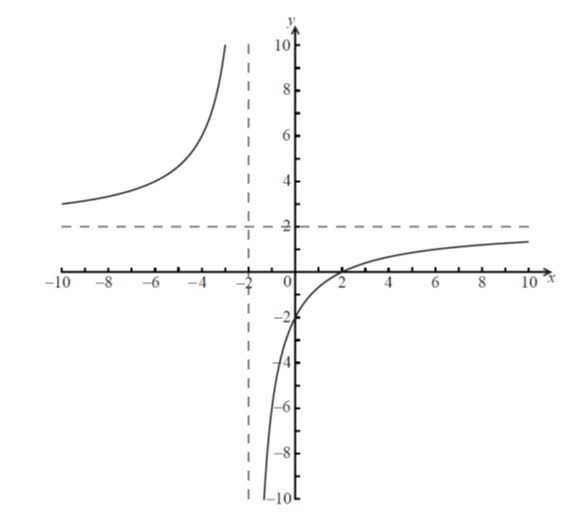(a)        Find the value of $a$  , the value of $b$  and the value of c .        [4marks]

(b) Using the values of  and $c$  found in part (a), sketch the graph of  $y=\left|\frac{b+cx}{a+x}\right|$ on the axes below, showing clearly all intercepts and asymptotes.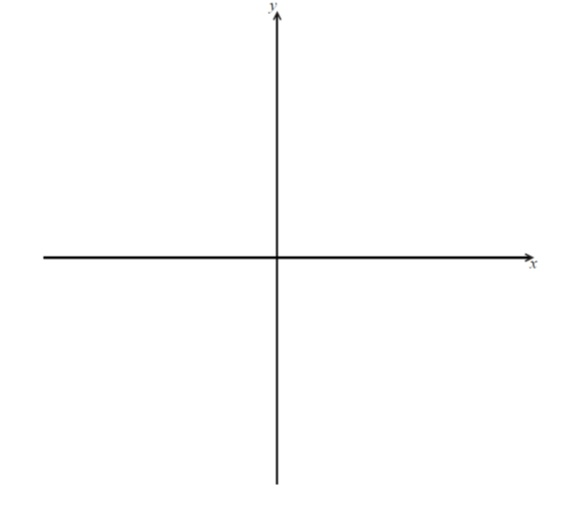Q8.   [M11.P1.TZ1]

The diagram below shows the graph of the function $y=f\left(x\right)$ , defined for all  $x\in \mathrm{ℝ}$ , where  $b>a>0$ .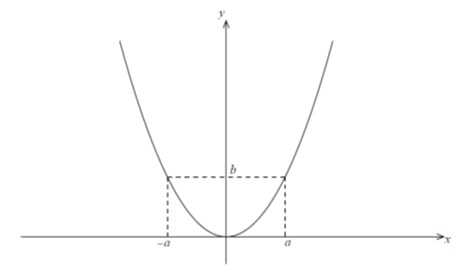Consider the function  $g\left(x\right)=\frac{1}{f\left(x–a\right)–b}$ .

(a)        Find the largest possible domain of the function $g$ .   [2 marks]

(b)       On the axes below, sketch the graph of $y=g\left(x\right)$ . On the graph, indicate any asymptotes and local maxima or minima, and write down their equations and coordinates.      [6 marks]Q9.   [M12.P1]

The graph of  $y=f\left(x\right)$ is shown below, where A is a local maximum point and D is a local minimum point.(a)        On the axes below, sketch the graph of  $y=\frac{1}{f\left(x\right)}$ , clearly showing the coordinates of the images of the points A, B and D, labelling them  and  respectively and the equations of any vertical asymptotes.    [3 marks]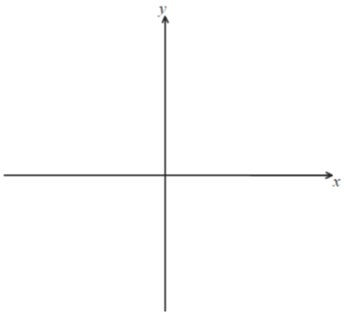Q10.   [M11.P1.TZ2]

The diagram shows the graph of $y=f\left(x\right)$  . The graph has a horizontal asymptote at $y=2$  .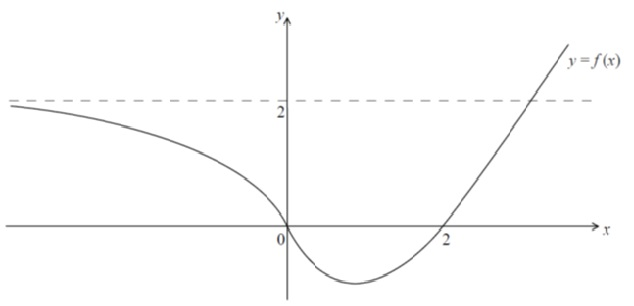(a)        Sketch the graph of  $y=f\left(x\right)$  .          [3 marks]

(b)       Sketch the graph of  $y=xf\left(x\right)$ .        [3marks]

Q11.   [M12.P1.TZ1]

The graphs of  $y=\left|x+1\right|$ and  $y=\left|x–3\right|$ are shown below.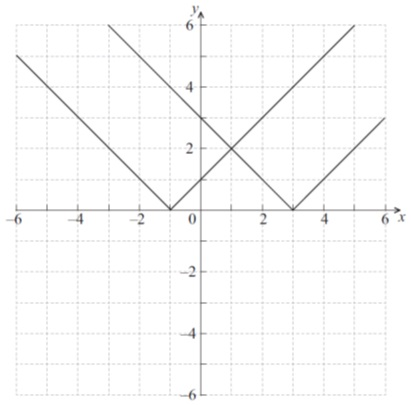Let  $f\left(x\right)=\left|x+1\right|–\left|x–3\right|$ .

(a)        Draw the graph of  $y=f\left(x\right)$  on the blank grid below.        [4 marks]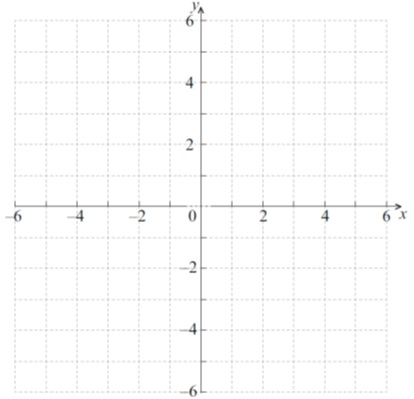Q12.   [M08.P2]

Let   $f\left(x\right)={x}^{3}+a{x}^{2}+bx+c$ , where  . The diagram shows the graph of $y=f\left(x\right)$  .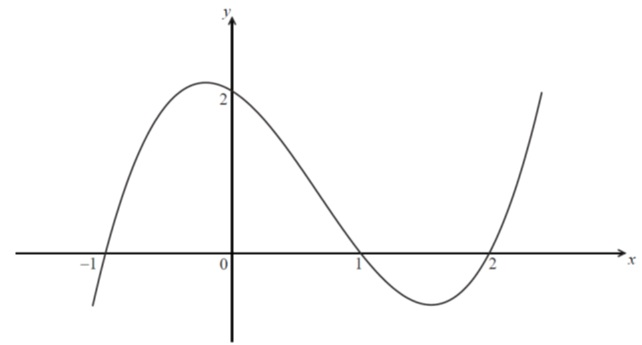(a)        Using the information shown in the diagram, find the values of  .             [4 marks]

(b)       If  ,

(i)        state the coordinates of the points where the graph of $g$ intercepts the x-axis.

(ii)       Find the y-intercept of the graph of g .   [3 marks]